# Class 10 Maths NCERT Solutions for Similar triangles EXERCISE 6.3

In this page we have Class 10 Maths NCERT Solutions for Similar triangles for EXERCISE 6.3 on pages 138,139,140 and 141. Hope you like them and do not forget to like , social_share and comment at the end of the page.
Formula Used in this exercise
(i) AA or AAA Similarity Criterion:
If all the angles or two angles are equal between two triangles, then Triangles are similar and corresponding sides are in proportion

(ii) SSS Similar Criterion:
If in two triangles, sides of one triangle is proportion to other triangle, then triangles are similar and corresponding angles will be equal between the triangles
(iii) SAS Similar Criterion:
If one angle is equal to one angle of another triangle and sides including those angles are proportional, the triangles are similar

## Triangles EXERCISE 6.3

Question 1
State which pairs of triangles in below figure are similar. Write the similarity criterion used by you for answering the question and write the pairs of similar triangles in the symbolic form: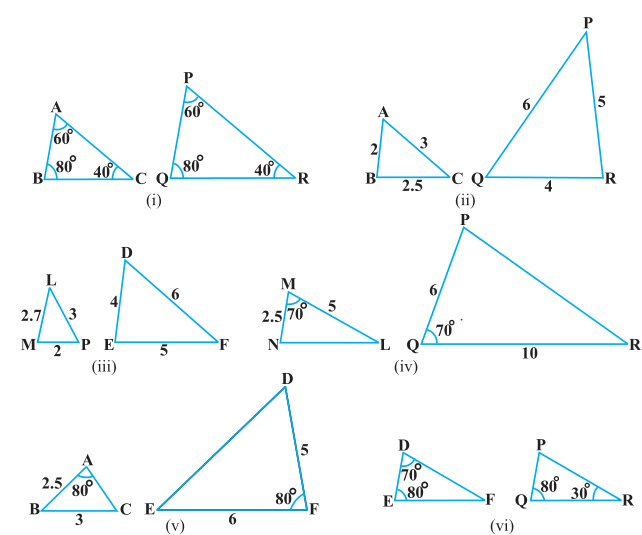(i) In ΔABC and ΔPQR, we have
∠A = ∠P = 60°
∠B = ∠Q = 80°
∠C = ∠R = 40°
So  as per AAA similarity criterion
We have ΔABC ~ ΔPQR

(ii) In ΔABC and ΔPQR, we have
AB/QR = BC/RP = CA/PQ= 1/2
So, SSS similarity criterion
We have ΔABC ~ ΔQRP

(iii) In ΔLMP and ΔDEF, we have
LM = 2.7, MP = 2, LP = 3, EF = 5, DE = 4, DF = 6
$\frac {PM}{DE} = \frac {2}{4} = \frac {1}{2}$
$\frac {PL}{DF} = \frac {3}{6} = \frac {1}{2}$
$\frac {LM}{EF}= \frac {2.7}{5} = \frac {27}{50}$
Here,$\frac {PM}{DE} =\frac {PL}{DF} \ne \frac {LM}{EF}$
Hence, ΔLMP and ΔDEF are not similar.

(iv) In ΔMNL and ΔQPR, we have
$\frac {MN}{QP} =\frac {2.5}{6}$
$\frac {ML}{QR} = \frac {1}{2}$
So,$\frac {MN}{QP} \ne \frac {ML}{QR}$ ∠M = ∠Q = 70°
Hence ΔMNL ~ ΔQPR are not similar

(v) In ΔABC and ΔDEF, we have
AB = 2.5, BC = 3, ∠A = 80°, EF = 6, DF = 5, ∠F = 80°
Here, $\frac {AB}{DF} = \frac {2.5}{5} = \frac {1}{2}$
And, $\frac {BC}{EF} = \frac {3}{6} = \frac {1}{2}$
But the included angle of the sides proportional is not equal
∠B ≠ ∠F
Hence, ΔABC and ΔDEF are not similar.

(vi) In ΔDEF, we have
Two angles given as 80 and 70, so third angle will
∠F = 180-70-80=30°
In PQR, we have
Two angles given as 80 and 30, so third angle will
∠P = 180-30-80=70°
In ΔDEF and ΔPQR, we have
∠D = ∠P = 70°
∠F = ∠Q = 80°
∠F = ∠R = 30°
By AAA similarity criterion
Hence, ΔDEF ~ ΔPQR

Question 2
In the below figure, ΔODC ~ ΔOBA, ∠ BOC = 125° and ∠ CDO = 70°. Find ∠ DOC, ∠ DCO and ∠ OAB.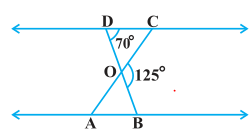Solution
DOB is a straight line.
Therefore, ∠DOC + ∠ COB = 180°
⇒ ∠DOC = 180° - 125°
= 55°
In ΔDOC,
Sum of the measures of the angles of a triangle is 180º
So ∠DCO + ∠ CDO + ∠ DOC = 180°
Therefore ∠DCO = 55°
It is given that ΔODC ~ ΔOBA.
Since Corresponding angles are equal in similar triangles
∠OAB = ∠OCD
So, ∠ OAB = 55°

Question 3
Diagonals AC and BD of a trapezium ABCD with AB || DC intersect each other at the point O. Using a similarity criterion for two triangles, show that AO/OC = OB/OD
Solution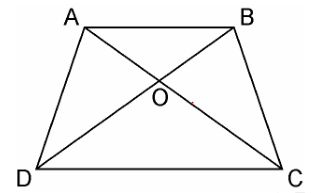In ΔDOC and ΔBOA,
Alternate interior angles are equal as AB || CD
∠CDO = ∠ABO
Alternate interior angles are equal as AB || CD
∠DCO = ∠BAO
Vertically opposite angles
∠DOC = ∠BOA

By AAA similarity criterion
ΔDOC ~ ΔBOA
Now Corresponding sides are proportional as similar triangles
$\frac {DO}{BO} = \frac {OC}{OA}$
Therefore, $\frac {OA}{OC} = \frac {OB}{OD}$

Question 4.
In the below figure, QR/QS = QT/PR and ∠1 = ∠2. Show that ΔPQS ~ ΔTQR.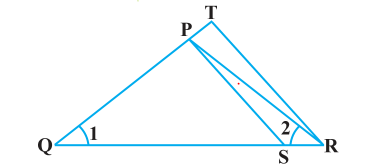Solution
In ΔPQR,
∠1 = ∠2
So, ∠PQR = ∠PRQ
Therefore opposite will be equal
PQ = PR ...(i)
Given
$\frac {QR}{QS} = \frac {QT}{PR}$
Using (i), we get
$\frac {QR}{QS} =\frac {QT}{QP}$ ...(ii)
In ΔPQS and ΔTQR,
$\frac {QR}{QS} =\frac {QT}{QP}$ [using (ii)]
∠Q = ∠Q
So  As  per SAS similarity criterion
ΔPQS ~ ΔTQR
Question 5.
S and T are point on sides PR and QR of ΔPQR such that ∠P = ∠RTS. Show that ΔRPQ ~ ΔRTS.
Solution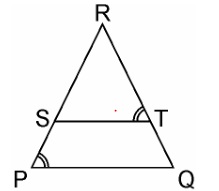In ΔRPQ and ΔRST,
∠RTS = ∠QPS (Given)
∠R = ∠R (Common angle)
As two angles are equal
∠RST = ∠RQP
By AAA similarity criterion
we get
ΔRPQ ~ ΔRTS

Question 6.
In the below figure, if ΔABE ≅ ΔACD, show that ΔADE ~ ΔABC.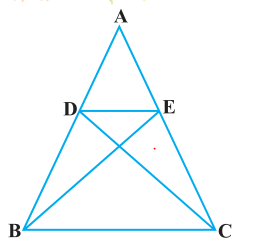Solution
It is given that ΔABE ≅ ΔACD.
By corresponding parts of the two congruent triangles
AB = AC   -(a)
Dividing equation (b) by (a)
∠A = ∠A [Common angle]
By SAS similarity criterion

Question 7
In the below figure, altitudes AD and CE of ΔABC intersect each other at the point P. Show that: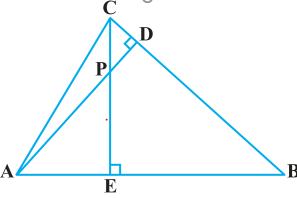(i) ΔAEP ~ ΔCDP
(ii) ΔABD ~ ΔCBE
(iv) ΔPDC ~ ΔBEC

Solution
(i) In ΔAEP and ΔCDP,
As Each 900   ∠AEP = ∠CDP
∠APE = ∠CPD (Vertically opposite angles)
As two angles are equal, third angle will also be equal
∠DCP = ∠PAE
By using AAA similarity criterion,
ΔAEP ~ ΔCDP
(ii) In ΔABD and ΔCBE,
∠ABD = ∠CBE (Common)
As two angles are equal, third angle will also be equal
∠DAB = ∠BCE
By using AAA similarity criterion,
ΔABD ~ ΔCBE
∠PAE = ∠DAB (Common)
As two angles are equal, third angle will also be equal
Hence, by using AAA similarity criterion,
(iv) In ΔPDC and ΔBEC,
∠PDC = ∠BEC (Each 90°)
∠PCD = ∠BCE (Common angle)
As two angles are equal, third angle will also be equal
Hence, by using AAA similarity criterion,
ΔPDC ~ ΔBEC

Question 8.
E is a point on the side AD produced of a parallelogram ABCD and BE intersects CD at F. Show that ΔABE ~ ΔCFB.
Solution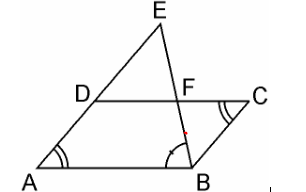In ΔABE and ΔCFB,
∠A = ∠C (Opposite angles of a parallelogram)
∠AEB = ∠CBF (Alternate interior angles as AE || BC)
So, two angles are equal so third angle would be also equal
∠ABE=∠BFC
By AAA similarity criterion
Therefore, ΔABE ~ ΔCFB

Question 9
In the below figure, ABC and AMP are two right triangles, right angled at B and M respectively, prove that: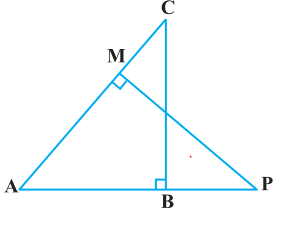(i) ΔABC ~ ΔAMP
(ii) $\frac {CA}{PA} = \frac {BC}{MP}$
Solution
(i) In ΔABC and ΔAMP, we have
∠A = ∠A (common angle)
∠ABC = ∠AMP = 90° (each 90°)
As two angles are equal, third angle will also be equal
By AAA similarity criterion
ΔABC ~ ΔAMP
(ii) As, ΔABC ~ ΔAMP
If two triangles are similar, then the corresponding sides are proportional
Hence, $\frac {CA}{PA} = \frac {BC}{MP}$

Question 10.
CD and GH are respectively the bisectors of ∠ACB and ∠EGF such that D and H lie on sides AB and FE of ΔABC and ΔEFG respectively. If ΔABC ~ ΔFEG, Show that:
(i) CD/GH = AC/FG
(ii) ΔDCB ~ ΔHGE
(iii) ΔDCA ~ ΔHGF
Solution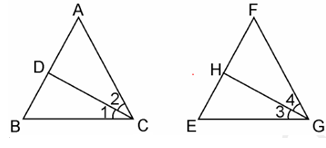(i) It is given that ΔABC ~ ΔFEG.

Therefore, all corresponding angles should be equals
∠A = ∠F, ∠B = ∠E, and ∠ACB = ∠FGE
Now as ∠ACB = ∠FGE, angle bisector are equals
∠ACD = ∠FGH
And, ∠DCB = ∠HGE
In ΔACD and ΔFGH,
∠A = ∠F
∠ACD = ∠FGH
By AA similarity criterion
Therefore ΔACD ~ ΔFGH
Now the corresponding sides are proportional
$\frac {CD}{GH} = \frac {AC}{FG}$
(ii) In ΔDCB and ΔHGE,
∠DCB = ∠HGE
∠B = ∠E
By AA similarity criterion
Therefore ΔDCB ~ ΔHGE
(iii) In ΔDCA and ΔHGF,
∠ACD = ∠FGH
∠A = ∠F
By AA similarity criterion
Therefore ΔDCA ~ ΔHGF

Question 11.
In the following figure, E is a point on side CB produced of an isosceles triangle ABC with AB = AC. If AD ⊥ BC and EF ⊥ AC, prove that ΔABD ~ ΔECF.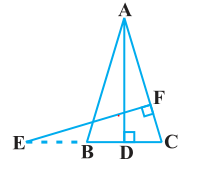Solution
It is given that ABC is an isosceles triangle.
Therefore,
AB = AC
So, ∠ABD = ∠ECF --(i)
Now in ΔABD and ΔECF,
And from equation (i)
∠ABD = ∠ECF
So, By using AA similarity criterion
ΔABD ~ ΔECF

Question 12.
Sides AB and BC and median AD of a triangle ABC are respectively proportional to sides PQ and QR and median PM of ΔPQR. Show that ΔABC ~ ΔPQR.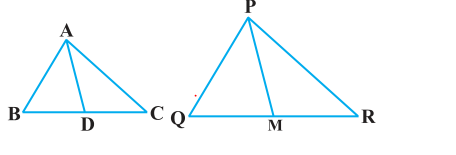Solution
Given: ΔABC and ΔPQR, AB, BC and median AD of ΔABC are proportional to sides PQ, QR and median PM of ΔPQR
i.e., AB/PQ = BC/QR = AD/PM
Now it can be written as
$\frac {AB}{PQ} = \frac {(1/2) BC}{ (1/2) QR} = \frac {AD}{PM}$
Or
$\frac {AB}{PQ} = \frac {BD}{QM} = \frac {AD}{PM}$ (D is the mid-point of BC. M is the midpoint of QR)
So, As per SSS similarity criterion
ΔABD ~ ΔPQM
Now we know that
Corresponding angles of two similar triangles are equal
So, ∠ABD = ∠PQM
⇒ ∠ABC = ∠PQR
Now In ΔABC and ΔPQR
$\frac {AB}{PQ} = \frac {BC}{QR}$ .
∠ABC = ∠PQR
By SAS similarity criterion
ΔABC ~ ΔPQR
Question 13.
D is a point on the side BC of a triangle ABC such that ∠ADC = ∠BAC. Show that CA2 = CB.CD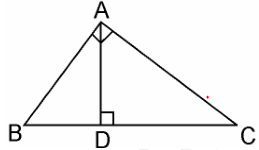∠ACD = ∠BCA (Common angle)
By AA similarity criterion
We know that corresponding sides of similar triangles are in proportion.
$\frac {CA}{CB} =\frac {CD}{CA}$
$CA^2= CB.CD$
Question 15.
A vertical pole of a length 6 m casts a shadow 4m long on the ground and at the same time a tower casts a shadow 28 m long. Find the height of the tower.
Length of the vertical pole = 6m (Given)
Shadow of the pole = 4 m (Given)
Let Height of tower = h m
Length of shadow of the tower = 28 m (Given)
In ΔABC and ΔDEF,
angular elevation of sun remains same
∠C = ∠E
∠B = ∠F = 90°
By AA similarity criterion ΔABC ~ ΔDEF
$\frac {AB}{DF} = \frac {BC}{EF}$ (If two triangles are similar corresponding sides are proportional)
$\frac {6}{h}= \frac {4}{28}$
h= 6×28/4
h=6 × 7
h= 42 m
Hence, the height of the tower is 42 m.

Question 16.
If AD and PM are medians of triangles ABC and PQR, respectively where ΔABC ~ ΔPQR prove that AB/PQ = AD/PM.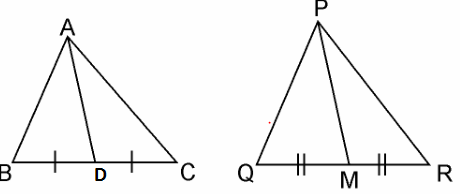It is given that ΔABC ~ ΔPQR
We know that the corresponding sides of similar triangles are in proportion.
$\frac {AB}{PQ} = \frac {AC}{PR} = \frac {BC}{QR}$ ...(i)
Also, ∠A = ∠P, ∠B = ∠Q, ∠C = ∠R …(ii)
Since AD and PM are medians, they will divide their opposite sides.
BD = BC/2 and QM = QR/2 ...(iii)
From equations (i) and (iii), we get
AB/PQ = BD/QM ...(iv)
In ΔABD and ΔPQM,
∠B = ∠Q [Using equation (ii)]
AB/PQ = BD/QM [Using equation (iv)]
By SAS Similarity
ΔABD ~ ΔPQM

## Summary

1. NCERT Solutions for Class 10 Maths: Chapter 6 triangles Exercise 6.3 has been prepared by Expert with utmost care. If you find any mistake.Please do provide feedback on mail.You can download this as pdf
2. This chapter 6 has total 5 Exercise 6.1 ,6.2,6.3 ,6.4 and 6.5. This is the Third exercise in the chapter.You can explore previous exercise of this chapter by clicking the link belowGo back to Class 10 Main Page using below links

### Practice Question

Question 1 What is $1 - \sqrt {3}$ ?
A) Non terminating repeating
B) Non terminating non repeating
C) Terminating
D) None of the above
Question 2 The volume of the largest right circular cone that can be cut out from a cube of edge 4.2 cm is?
A) 19.4 cm3
B) 12 cm3
C) 78.6 cm3
D) 58.2 cm3
Question 3 The sum of the first three terms of an AP is 33. If the product of the first and the third term exceeds the second term by 29, the AP is ?
A) 2 ,21,11
B) 1,10,19
C) -1 ,8,17
D) 2 ,11,20